# Maths - Geometry - Further ReadingCurves and Surfaces in Geometric Modeling: Theory and AlgorithmsIntroduction to Topological Manifolds (Graduate Texts in Mathematics S.)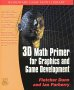3D Math Primer - Aimed at complete beginners to vector and matrix algebra.The NURBs Book (Monographs in Visual Communication) - A NURBS book for software developer, it covers the theory and also the practical side.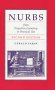NURBS: from Projective Geometry to Practical Use - This is more useful for learning the mathematical foundations of NURBS than for programmers looking for a reference book on practical algorithms or applications of NURBS.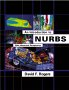An Introduction to NURBS: With Historical Perspective - This is a good textbook for the theory and maths of curve and surface computer modeling.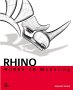Rhino NURBS 3D Modelling. Rhino is a 3D Modelling program, this book explains how to use it.Schaum's Outline of Theory and Problems of Tensor Calculus - I'm finding this hard going, it starts off with as review of linear algebra, matrix notation, etc. It redefines a lot of conventions which are hard to relearn, such as superscrips instead of subscripts to identify elements, and a summation convention, then it goes into coordinate transformations. I cant find any definition of what a tensor is. I think this book is aimed at people who already have some knowledge of the subject.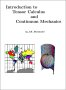Introduction to Tensor Calculus and Continuum Mechanics